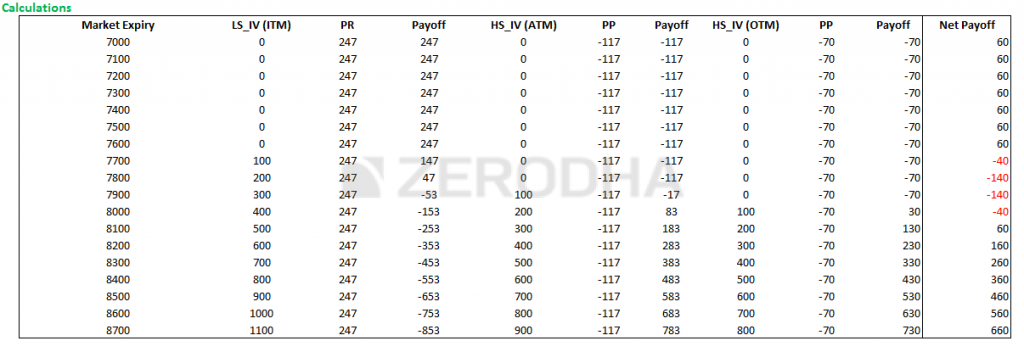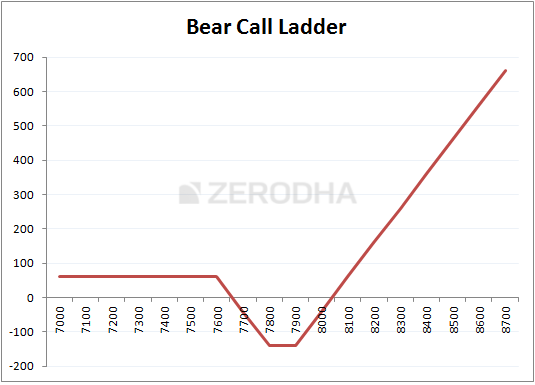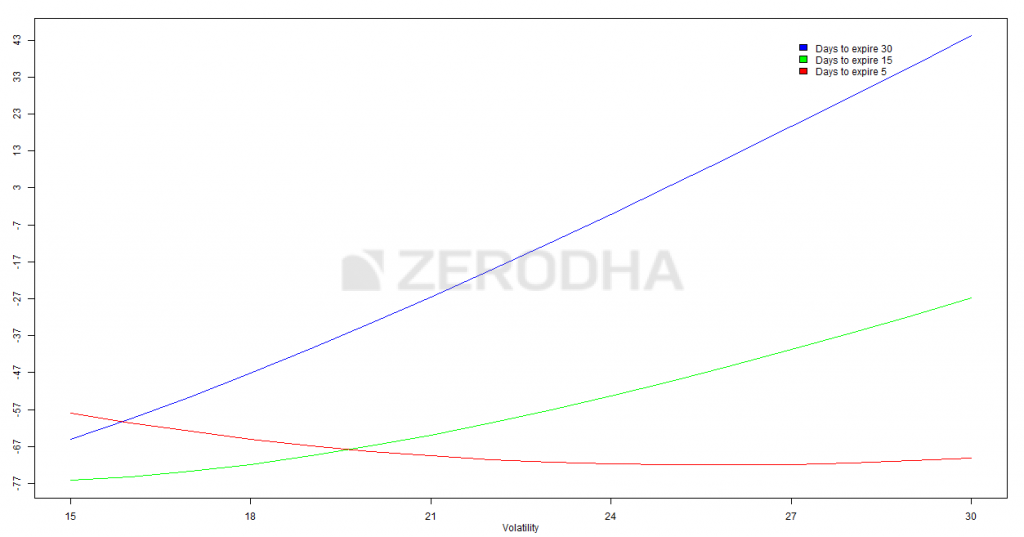## 5.1 – Background

The ‘Bear’ in the “Bear Call Ladder” should not deceive you to believe that this is a bearish strategy. The Bear Call Ladder is an improvisation over the Call ratio back spread; this clearly means you implement this strategy when you are out rightly bullish on the stock/index.

In a Bear Call Ladder, the cost of purchasing call options is financed by selling an ‘in the money’ call option. Further, the Bear Call Ladder is also usually setup for a ‘net credit’, where the cash flow is invariably better than the cash flow of the call ratio back spread. However, do note that both these strategies showcase similar payoff structures but differ slightly in terms of the risk structure.

## 5.2 – Strategy Notes

The Bear Call Ladder is a 3 leg option strategy, usually setup for a “net credit”, and it involves –

1. Selling 1 ITM call option
2. Buying 1 ATM call option
3. Buying 1 OTM call option

This is the classic Bear Call Ladder setup, executed in a 1:1:1 combination. The bear Call Ladder has to be executed in the 1:1:1 ratio meaning for every 1 ITM Call option sold, 1 ATM and 1 OTM Call option has to be bought. Other combination like 2:2:2 or 3:3:3 (so on and so forth) is possible.

Let’s take an example – assume Nifty Spot is at 7790 and you expect Nifty to hit 8100 by the end of expiry. This is clearly a bullish outlook on the market. To implement the Bear Call Ladder –

1. Sell 1 ITM Call option
2. Buy 1 ATM Call option
3. Buy 1 OTM Call option

Make sure –

1. The Call options belong to the same expiry
2. Belongs to the same underlying
3. The ratio is maintained

The trade set up looks like this –

1. 7600 CE, one lot short, the premium received for this is Rs.247/-
2. 7800 CE, one lot long, the premium paid for this option is Rs.117/-
3. 7900 CE, one lot long, the premium paid for this option is Rs.70/-
4. The net credit would be 247-117-70 = 60

With these trades, the bear call ladder is executed. Let us check what would happen to the overall cash flow of the strategies at different levels of expiry.

Do note we need to evaluate the strategy payoff at various levels of expiry as the strategy payoff is quite versatile.

Scenario 1 – Market expires at 7600 (below the lower strike price)

We know the intrinsic value of a call option (upon expiry) is –

Max [Spot – Strike, 0]

The 7600 would have an intrinsic value of

Max [7600 – 7600, 0]

= 0

Since we have sold this option, we get to retain the premium received i.e Rs.247/-

Likewise the intrinsic value of 7800 CE and 7900 CE would also be zero; hence we lose the premium paid i.e Rs.117 and Rs.70 respectively.

= 247 – 117 – 70

= 60

Scenario 2 – Market expires at 7660 (lower strike + net premium received)

The 7600 CE would have an intrinsic value of –

Max [Spot – Strike, 0]

The 7600 would have an intrinsic value of

Max [7660 – 7600, 0]

= 60

Since the 7600 CE is short, we will lose 60 from 247 and retain the balance

= 247 – 60

= 187

The 7800 and 7900 CE would expire worthless, hence we lose the premium paid i.e 117 and 70 respectively.

The total strategy payoff would be –

= 187 – 117 – 70

= 0

Hence at 7660, the strategy would neither make money nor lose money. Hence this is considered a (lower) breakeven point.

Scenario 3 – Market expires at 7700 (between the breakeven point and middle strike i.e 7660 and 7800)

The intrinsic value of 7600 CE would be –

Max [Spot – Strike, 0]

= [7700 – 7600, 0]

= 100

Since, we have sold this option for 247 the net pay off from the option would be

247 – 100

= 147

On the other hand we have bought 7800 CE and 7900 CE, both of which would expire worthless, hence we lose the premium paid for these options i.e 117 and 70 respectively –

Net payoff from the strategy would be –

147 – 117 – 70

= – 40

Scenario 4 – Market expires at 7800 (at the middle strike price)

Pay attention here, as this is where the tragedy strikes!

The 7600 CE would have an intrinsic value of 200, considering we have written this option for a premium of Rs.247, we stand to lose the intrinsic value which is Rs.200.

Hence on the 7600 CE, we lose 200 and retain –

247 – 200

= 47/-

Both 7800 CE and 7900 CE would expire worthless, hence the premium that we paid goes waste, i.e 117 and 70 respectively. Hence our total payoff would be –

47 – 117 – 70

= -140

Scenario 5 – Market expires at 7900 (at the higher strike price)

Pay attention again, tragedy strikes again ☺

The 7600 CE would have an intrinsic value of 300, considering we have written this option for a premium of Rs.247, we stand to lose all the premium value plus more.

Hence on the 7600 CE, we lose –

247 – 300

= -53

Both 7800 CE would have an intrinsic value of 100, considering we have paid a premium of Rs.117, the pay off for this option would be –

100 – 117

= – 17

Finally 7900 CE would expire worthless, hence the premium paid i.e 70 would go waste. The final strategy payoff would be –

-53 – 17 – 70

= -140

Do note, the loss at both 7800 and 7900 is the same.

Scenario 6 – Market expires at 8040 (sum of long strike minus short strike minus net premium)

Similar to the call ratio back spread, the bear call ladder has two breakeven points i.e the upper and lower breakeven. We evaluated the lower breakeven earlier (scenario 2), and this is the upper breakeven point. The upper breakeven is estimated as –

(7900 + 7800) – 7600 – 60

= 15700 – 7600 – 60

= 8100 – 60

= 8040

Do note, both 7900 and 7800 are strikes we are long on, and 7600 is the strike we are short on. 60 is the net credit.

So at 8040, all the call options would have an intrinsic value –

7600 CE would have an intrinsic value of 8040 – 7600 = 440, since we are short on this at 247, we stand to lose 247 – 440 = -193.

7800 CE would have an intrinsic value of 8040 – 7800 = 240, since we are long on this at 117, we make 240 – 117 = +123

7900 CE would have an intrinsic value of 8040 – 7900 = 140, since we are long on this at 70, we make 140 – 70 = +70

Hence the total payoff from the Bear Call Ladder would be –

-193 + 123 + 70

= 0

Hence at 8040, the strategy would neither make money nor lose money. Hence this is considered a (upper) breakeven point.

Do note, at 7800 and 7900 the strategy was making a loss and at 8040 the strategy broke even. This should give you a sense that beyond 8040, the strategy would make money. Lets just validate this with another scenario.

Scenario 7 – Market expires at 8300

At 8300 all the call options would have an intrinsic value.

7600 CE would have an intrinsic value of 8300 – 7600 = 700, since we are short on this at 247, we stand to lose 247 – 700 = -453.

7800 CE would have an intrinsic value of 8300 – 7800 = 500, since we are long on this at 117, we make 500 – 117 = +383

7900 CE would have an intrinsic value of 8300 – 7900 = 400, since we are long on this at 70, we make 400 – 70 = +330

Hence the total payoff from the Bear Call Ladder would be –

-453 + 383 + 330

= 260

As you can imagine, the higher the market move, the higher is the profit potential. Here is a table that gives you the payoffs at various levels.Do notice, when the market goes below you stand to make a modest gain of 60 points, but when the market moves up the profits are uncapped.

## 5.3 – Strategy Generalization

Going by the above discussed scenarios we can make few generalizations –

• Spread = technically this is a ladder and not really a spread. However the 1st two option legs creates a classic “spread” wherein we sell ITM and buy ATM. Hence the spread could be taken as the difference between the ITM and ITM options. In this case it would be 200 (7800 – 7600)
• Net Credit = Premium Received from ITM CE – Premium paid to ATM & OTM CE
• Max Loss = Spread (difference between the ITM and ITM options) – Net Credit
• Max Loss occurs at = ATM and OTM  Strike
• The payoff when market goes down = Net Credit
• Lower Breakeven = Lower Strike + Net Credit
• Upper Breakeven = Sum of Long strike minus short strike minus net premium

Here is a graph that highlights all these important points –Notice how the strategy makes a loss between 7660 and 8040, but ends up making a huge profit if the market moves past 8040. Even if the market goes down you still end up making a modest profit. But you are badly hit if the market does not move at all. Given this characteristics of the Bear Call Ladder, I would suggest you implement the strategy only when you are absolutely sure that the market will move, irrespective of the direction.

From my experience, I believe this strategy is best executed on stocks (rather than index) when the quarterly results are due.

## 5.4 – Effect of Greeks

The effect of Greeks on this strategy is very similar to the effect of Greeks on Call Ratio Back spread, especially the volatility bit. For your easy reference, I’m reproducing the discussion on volatility we had in the previous chapter.There are three colored lines depicting the change of “net premium” aka the strategy payoff versus change in volatility. These lines help us understand the effect of increase in volatility on the strategy keeping time to expiry in perspective.

1. Blue Line – This line suggests that an increase in volatility when there is ample time to expiry (30 days) is beneficial for the Bear Call Ladder spread. As we can see the strategy payoff increases from -67 to +43 when the volatility increase from 15% to 30%. Clearly this means that when there is ample time to expiry, besides being right on the direction of stock/index you also need to have a view on volatility. For this reason, even though I’m bullish on the stock, I would be a bit hesitant to deploy this strategy at the start of the series if the volatility is on the higher side (say more than double of the usual volatility reading)
2. Green line – This line suggests that an increase in volatility when there are about 15 days time to expiry is beneficial, although not as much as in the previous case. As we can see the strategy payoff increases from -77 to -47 when the volatility increase from 15% to 30%.
3. Red line – This is an interesting, counter intuitive outcome. When there are very few days to expiry, increase in volatility has a negative impact on the strategy! Think about it, increase in volatility when there are few days to expiry enhances the possibility of the option to expiry OTM, hence the premium decreases. So, if you are bullish on a stock / index with few days to expiry, and you also expect the volatility to increase during this period then thread cautiously.

### Key takeaways from this chapter

1. Bear Call Ladder is an improvisation over the Call Ratio Spread
2. Invariably the cost of executing a bear call ladder is better than the Call Ratio Spread, but the range above which the market has to move also becomes large
3. The Bear Call Ladder is executed by selling 1 ITM CE, buying 1 ATM CE, and 1 OTM CE
4. Net Credit = Premium Received from ITM CE – Premium paid to ATM & OTM CE
5. Max Loss = Spread (difference between the ITM and ITM options) – Net Credit
6. Max Loss occurs at = ATM and OTM  Strike
7. The payoff when market goes down = Net Credit
8. Lower Breakeven = Lower Strike + Net Credit
9. Upper Breakeven = Sum of Long strike minus short strike minus net premium
10. Execute the strategy only when you are convinced that the market will move significantly higher.

1.Ajay says:

The best time to implement this strategy is during the first 15 days of the month, 4-5 days before the announcement of Quarterly results of a company, just before the volatility picks up so that the pay off increases due to the increase in volatility in the following days?

Also, when would be the right time to exit from the strategy? Once the results are announced and the stock reacts or on expiry?

•Karthik Rangappa says:

Ajay – yes increase in volatility (especially in the first half of the series) is great for this strategy as this tends to lift the payoffs to the positive territory.

If you are playing this for results, exit the positions after the result announcement – once the directional movement picks up.

•Guruprasad V says:

“In a Bear Call Ladder, the cost of purchasing call options is financed by selling an ‘in the money’ call option.” what is the meaning for this line.

•Karthik Rangappa says:

It means, when you sell options you receive premium money, you can use this money to buy another option.

•Deep Dave says:

Hi,

When you refer to Volatility, do you refer to the Stocks Volatility or Indexs Volatility?

•Karthik Rangappa says:

Depends on the asset, if you are dealing a stock, then you need to look stock’s volatility. Likewise with Index.

2.SUNIL HC says:

How can we always in the profit zone while we option trading,?

•Karthik Rangappa says:

By being right all the time 🙂

3.Sunil HC says:

If the market in the bearish trend it is better to sell one lot ITM CE. and Buying one lot ATM CE. I think that enough to make money. Why we should buy one OTM call option. Plz clarify that sir

•Karthik Rangappa says:

You could try that, in fact I would encourage you to plot the individual payoff and check how it works. This will be a practice for you!

4.Sunil HC says:

Sir will you explain that how can we gaze the market trends for next days. What are the technicals can support to find out the marker way. Sir

•Karthik Rangappa says:

Suggest you read the module on Technical Analysis – http://zerodha.com/varsity/module/technical-analysis/ . You will get many pointers here.

5.Khyati Verdhan says:

Hi kartik
Can you give me a recipe of technical indicators to good enough, because it is very difficult and confusing to apply many indicators???

•Karthik Rangappa says:

I would suggest you look at candlestick patterns rather than indicators.